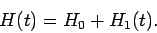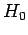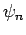Next: Preliminary Analysis Up: Time-Dependent Perturbation Theory Previous: Time-Dependent Perturbation Theory

# Introduction

Consider a system whose Hamiltonian can be written(1017)

Here,is again a simple time-independent Hamiltonian whose eigenvalues and eigenstates are known exactly. However,now represents a small time-dependent external perturbation. Let the eigenstates oftake the form(1018)

We know (see Sect. 4.12) that if the system is in one of these eigenstates then, in the absence of an external perturbation, it remains in this state for ever. However, the presence of a small time-dependent perturbation can, in principle, give rise to a finite probability that if the system is initially in some eigenstateof the unperturbed Hamiltonian then it is found in some other eigenstate at a subsequent time (sinceis no longer an exact eigenstate of the total Hamiltonian). In other words, a time-dependent perturbation can cause the system to make transitions between its unperturbed energy eigenstates. Let us investigate this effect.

Richard Fitzpatrick 2010-07-20